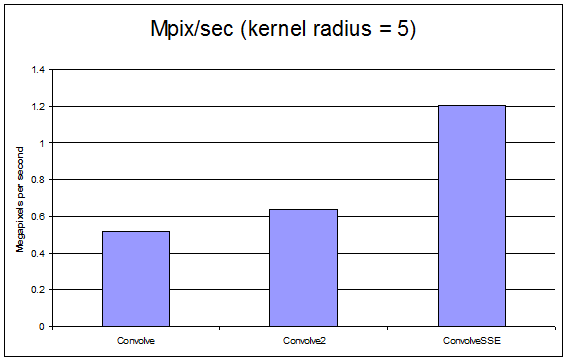## Advanced Image Processing with SSE

Pages: 1 2

In a previous article about image processing with SSE, we used some basic SSE intrinsics to perform a very easy image manipulation routine, removing all blue from an image. This task was easy, since each pixel was 8 bits per component, with 4 components (ARGB). However, for more advanced image processing functions such as 2D convolution, it is preferable to work with each color component as a 32-bit floating point number rather than an 8-bit unsigned integer.

## Image formats for SSE

SSE was originally developed with floating point operations in mind. Most integer SSE instructions came with the introduction to SSE2. Floating point numbers are preferable for many image processing algorithms because they offer the greatest amount of flexibility and accuracy. While most images are in a format with 8-bits per color component, before using SSE for more advanced algorithms, we will need to convert the entire image to 32-bits per color component. Because SSE operates on a 128-bit wide vector, we can fit 4 color components into a single SSE vector. So if our image has four color channels including alpha, each pixel is represented in a single SSE vector. Code for converting an image into a format more acceptable for SSE processing is below. Of course, this code may vary slightly depending on what framework you’re using for handling bitmaps. The code here works on GDI+. It is not computationally complex, and can even be sped up easily by OpenMP.

```int nPixels = height*bitmapData.Stride/4;
#pragma omp parallel
for (int i=0; i < nPixels; i++)
{
unsigned int curPixel = pRawBitmapOrig[i];
float alpha = (float)((curPixel & 0xff000000) >> 24);
float red = (float)((curPixel & 0x00ff0000) >> 16);
float green = (float)((curPixel & 0x0000ff00) >> 8);
float blue = (float)(curPixel & 0x000000ff);
pBitmapCopy[i*4] = alpha;
pBitmapCopy[i*4+1] = red;
pBitmapCopy[i*4+2] = green;
pBitmapCopy[i*4+3] = blue;
}```

## Convert beforehand, or on the fly?

In this tutorial, we will be doing a simple 2D convolution. Because each pixel is processed multiple times during convolution, it actually faster for SSE and non-SSE code alike to convert the image to a 32-bit floating point  per channel format once rather than on the fly.

## Why SSE for image processing?

Typically, each color component of an image is processed separately, and identically. That means that we need to perform the exact same things four times since there are four color channels. SSE allows us to treat each pixel as one vector, so we can cut down on the number of separate math operations we have to do by four. This provides a great boost in productivity, especially in math heavy operations such as convolution. Since all modern x86 processors support the SSE instruction set, we can be confident that our code will work on the overwhelming majority of computers. A quick snippet of code below shows how SSE intrinsics are used for SSE floating point math operations. Full code can be found on the next page.

```float totalWeight = 0;
__m128 totalColor = _mm_setzero_ps(); // Reset total color vector to all zeros.
{
if (k < 0 || k >= height) continue;
{
if (l<0 || l>= width) continue;
int base = k*bitmapData.Stride + l*4;
float diff;
if (i==k && j==l) diff = 1.0f;
else
diff = 1.0f/(abs(k-i) + abs(l-j));
__m128 curColor = _mm_loadu_ps(&pBitmapCopy[base]); // Load the pixel into an SSE vector
__m128 diffVector = _mm_set_ps1(diff); // Set the current weight of kernel to all 4 floats in vector
__m128 resultAddition = _mm_mul_ps(curColor, diffVector); // multiply the color channels by kernel weightHere, we have three easy to read examples of convolution. The results are discussed here, and the code can be found on the next page. If you’re looking for some example SSE code, feel free to go to the next page. The first is the simplest code, and converts integer color components to floating point on the fly. Next, we allocate 4 times as much memory to hold a temporary bitmap in a format with 32-bit floating point numbers for each color channel per pixel. This change resulted in a healthy 23% performance increase compared to the original, which is great. Finally, we have the same code, but using SSE intrinsics to perform most of the math operations. This results in code which is 133% faster than the original code! This represents the true power of SSE when doing mathematically intensive image manipulation. This benchmark was run with a kernel radius of 5, which means each pixel needs 484 multiplications and another 484 additions, which can be time consuming especially for large images. These results were performed using an Intel i7-930 processor at 2.8 GHz. While this performance could never match that of a CUDA or OpenCL capable device, SSE is something you can depend on everyone having inside their personal computer.